# Team:KU Leuven/Modeling/Hybrid

$C$: concentration [nmol/cl]
$\vec{r}$: position vector [µm]
$t$: time [h]
$D$: diffusion coefficient [µm^2/h]

$C$: concentration [nmol/cl]
$\vec{r}$: position vector [µm]
$t$: time [h]
$\alpha$: production constant [nmol/h]
$\rho_A$: density of type A bacteria [#/cl]

$C$: concentration [nmol/cl]
$\vec{r}$: position vector [µm]
$t$: time [h]
$k$: degradation constant [1/h]

$C$: concentration [nmol/cl]
$\vec{r}$: position vector [µm]
$t$: time [h]
$D$: diffusion coefficient [µm^2/h]
$\alpha$: production constant [nmol/h]
$\rho_A$: density of type A bacteria [#/cl]
$k$: degradation constant [1/h]

$\vec{r}$: position vector [µm]
$t$: time [h]
$\vec{F}_{applied}$: applied force [mN]
$\gamma$: frictional coefficient [mN*h/µm]

$\vec{r}$: position vector [µm]
$t$: time [h]
$\chi$: chemotactic sensitivity [µm^2*cl/(h*nmol)]
$S$: chemoattractant concentration [nmol/cl]
$\mu$: bacterial diffusion coefficient [µm^2/h]
$\vec{W}$: Wiener process [h^(1/2)]
$\kappa$: chemotactic sensitivity constant [-]

$n$: bacteria density [#/cl]
$\vec{r}$: position vector [µm]
$t$: time [h]
$\mu$: bacterial diffusion coefficient [µm^2/h]
$\chi$: chemotactic sensitivity [µm^2*cl/(h*nmol)]
$S$: chemoattractant concentration [nmol/cl]
$\kappa$: chemotactic sensitivity coefficient [-]

$\chi$: chemotactic sensitivity [µm^2*cl/(h*nmol)]
$S$: chemoattractant concentration [nmol/cl]
$\mu$: bacterial diffusion coefficient [µm^2/h]
$\kappa$: chemotactic sensitivity constant [-]
$S$: chemoattractant concentration [nmol/cl]
$\vec{r}$: position vector [µm]
$t$: time [h]

$E_p$: interaction potential [nJ]
$r_{ij}$: intercellular distance [µm]
$k$: “spring constant” [mN/µm]
$R_{cutoff}$: cutoff intercellular distance [µm]
$R_0$: equilibrium intercellular distance [µm]
$C$: constant to ensure continuity [nJ]

$C_1=-\frac{1}{2}\cdot k_3 \cdot(R_{cutoff}-R_0)^2$
$C_2=-\frac{1}{2}\cdot k_1 \cdot(\frac{R_0}{2}-\frac{k_1+k_2}{k_1}\cdot \frac{R_0}{2})^2 \\ +\frac{1}{2}\cdot k_2 \cdot(\frac{R_0}{2}-R_0)^2 +C_1$

$\vec{F}_i$: cell-cell force acting on cell i [mN]
$E_p$: interaction potential [nJ]
$r_{ij}$: intercellular distance [µm]
$\vec{e}_{ij}$: unit vector from cell i to cell j [-]
$k$: “spring constant” [mN/µm]
$R_{cutoff}$: cutoff intercellular distance [µm]
$R_0$: equilibrium intercellular distance [µm]

$\vec{r}$: position vector [µm]
$t$: time [h]
$\mu$: bacterial diffusion coefficient [µm^2/h]
$H$: concentration of AHL [nmol/cl]
$\vec{W}$: Wiener process [h^(1/2)]
$\gamma$: frictional coefficient [mN*h/µm]
$E_p$: interaction potential [nJ]
$r_{ij}$: intercellular distance [µm]
$\vec{e}_{ij}$: unit vector from cell i to cell j
$\chi$: chemotactic sensitivity [µm^2*cl/(h*nmol)]
$L$: concentration of leucine [nmol/cl]

$\chi$: chemotactic sensitivity [µm^2*cl/(h*nmol)]
$L$: concentration of leucine [nmol/cl]
$H$: concentration of AHL [nmol/cl]
$\mu$: bacterial diffusion coefficient [µm^2/h]
$\kappa$: chemotactic sensitivity constant [-]
$\vec{r}$: position vector [µm]
$t$: time [h]

$\mu$: bacterial diffusion coefficient [µm^2/h]
$H$: concentration of AHL [nmol/cl]
$\vec{r}$: position vector [µm]
$t$: time [h]

$K$: probability density [-]
$x$: stochastic variable [-]

$K_h$: kernel density [#/µm]
$x$: position [µm]
$h$: bandwidth [µm]

$\rho$: agent density [#/cl]
$\beta$: conversion factor [µm/cl]
$N$: number of agents [#]
$K_h$: kernel density [#/µm]
$x$: position [µm]
$x_i$: position of ith agent [µm]

Conversion factor $\beta$ is needed to express the 1-D density [#/µm] in units used in the PDE model [#/cl]. For the 2-D KDE $\beta$ has units [µm^2/cl]. The value of $\beta$ depends on assumptions made about the dimensions of the physical domain which are not modeled. For example, when modeling a 2-D virtual domain this constant will be high if bacteria are densely distributed along the height of the experimental petri dish.

$f$: function, e.g. concentration [nmol/cl]
$\hat{f}$: interpolated function, e.g. [nmol/cl]
$x$: independent variable, e.g. position [µm]
$x_i$: independent variable for ith measurement, e.g. [µm]

$f$: function, e.g. concentration [nmol/cl]
$\hat{f}$: interpolated function, e.g. [nmol/cl]
$x$: independent variable, e.g. position [µm]
$x_i$: independent variable for measurement (i,j), e.g. [µm]
$y$: independent variable, e.g. position [µm]
$y_j$: independent variable for measurement (i,j), e.g. [µm]
$Q_{i,j}$: symbol for grid point corresponding to measurement (i,j)

$f$: function, e.g. concentration [nmol/cl]
$\hat{f}$: interpolated function, e.g. [nmol/cl]
$x$: independent variable, e.g. position [µm]
$x_i$: independent variable for measurement (i,j), e.g. [µm]
$y$: independent variable, e.g. position [µm]
$y_j$: independent variable for measurement (i,j), e.g. [µm]
$Q_{i,j}$: grid point corresponding to measurement (i,j) [-]

$Re$: Reynolds number [-]
$\rho$: mass density [kg/m^3]
$v$: speed [m/s]
$L$: characteristic length [m]
$\eta$: dynamic viscosity [Pa*s]

$F$: force [N]
$m$: mass [kg]
$x$: position [m]
$t$: time [s]
$\eta$: dynamic viscosity [Pa*s]
$a$: radius of particle [m]
$v$: speed [m/s]

Stokes' law states that the force of viscosity for a spherical object moving through a viscous fluid is given by $F=6\pi\eta av$ with $a$ the radius of the sphere. Importantly, this force is proportional to the velocity $v$.

$v$: speed [m/s]
$v_0$: initial speed [m/s]
$\eta$: dynamic viscosity [Pa*s]
$a$: radius of cell [m]
$m$: mass [kg]
$t$: time [s]
$t_0$: start time [s]

To calculate the mass of a bacterium, we assume that the bacterium is spherical with radius $a$ and the density is twice that of water. Therefore, $m=4/3 \cdot \pi \cdot a^3 \cdot (2 \rho)$.

$\mu$: bacterial diffusion coefficient [µm^2/h]
$\vec{W}$: Wiener process [h^(1/2)]
$\Delta t$: timestep [h]
$\vec{N}$: normal distribution [-]

$\vec{N}$ indicates a vector of which the components are independent random variables that are normally distributed.

$\mu$: bacterial diffusion coefficient [µm^2/h]
$\Delta t$: timestep [h]
$\vec{N}$: normal distribution [-]

$X_1$: coordinate at next timestep [µm]
$x_1$: coordinate at current timestep [µm]
$\mu$: bacterial diffusion coefficient [µm^2/h]
$\Delta t$: timestep [h]
$N$: normal distribution [-]

$R$: intercellular distance at next timestep [µm]
$X_1$: coordinate at next timestep [µm]

$X_1$: coordinate at next timestep [µm]
$x_1$: coordinate at current timestep [µm]
$\mu$: bacterial diffusion coefficient [µm^2/h]
$\Delta t$: timestep [h]
$N$: normal distribution [-]

$\lambda$: noncentrality parameter [-]
$x_1$: coordinate at current timestep [µm]
$\mu$: bacterial diffusion coefficient [µm^2/h]
$\Delta t$: timestep [h]
$r_0$: intercellular distance at current timestep [µm]

$\bar{R}$: normalized intercellular distance at next timestep [µm]
$X_1$: coordinate at current timestep [µm]
$\mu$: bacterial diffusion coefficient [µm^2/h]
$\Delta t$: timestep [h]
$\chi^2$: chi-squared distribution [-]
$r_0$: intercellular distance at current timestep [µm]
$\lambda$: noncentrality parameter [-]

$\chi^2(k,\lambda)$ is the noncentral chi-squared distribution with $k$ degrees of freedom and noncentrality parameter $\lambda$.

$F$: cumulative probability function, returns the probability that $\bar{R}^2$ is smaller than a given $\bar{R^*}^2$ [-]
$\bar{R^*}$: given scaled and squared intercellular distance for which the cumulative probability function is calculated [µm]
$P$: probability [-]
$\bar{R}$: normalized intercellular distance at next timestep [µm]

Note that $F$ is defined with respect to the random variable $\bar{R}^2$ whose distribution is given in (23). Therefore, $F$ depends on the same parameters as $\chi^2$, which are the number of degrees of freedom $k$ and noncentrality parameter $\lambda = \frac{r^{2}_{0}}{4 \cdot \mu \cdot \Delta t}$.

$F^{-1}$: inverse cumulative probability function, returns $\bar{R^*}^2$ that $\bar{R}^2$ will not exceed with given probability $P$ [-]
$P^-$: desired probability of intercellular distance at next timestep exceeding the cutoff distance, $1-P^-$ gives the probability that it will not be greater [-]
$\bar{r}_{cutoff}$: at distances greater than this cutoff distance the cell no longer interact [µm]
$\mu$: bacterial diffusion coefficient [µm^2/h]
$\Delta t$: timestep [h]

$x'$: coordinate after enforcing periodic boundary conditions [µm]
$x$: coordinate before enforcing periodic boundary conditions [µm]
$L_x$: domain length [µm]

$D$: diffusion coefficient [µm^2/h]
$\Delta t$: timestep [h]
$\Delta x$: distance between grid points [µm]

$C$: concentration [nmol/cl]
$\vec{r}$: position vector [µm]
$t$: time [h]
$D$: diffusion coefficient [µm^2/h]
$\alpha$: production constant [nmol/h]
$\rho_A$: density of type A bacteria [#/cl]
$k$: degradation constant [1/h]

$C$: concentration [nmol/cl]
$n$: current step [-]
$D$: diffusion coefficient [µm^2/h]
$\delta^2_x$: second order finite difference operator [-]
$\alpha$: production constant [nmol/h]
$\rho_A$: density of type A bacteria [#/cl]
$\bar{n}$: indicates average over $[n; n+1]$ [h]
$k$: degradation constant [1/h]

For ease of notation we indicate values at time $t$ as the $n$th step in our solution. Similarly, $n+1/2$ and $n+1$ correspond to $t+1/2 \cdot \Delta t$ and $t+\Delta t$ respectively.

$r$: helper variable [-]
$D$: diffusion coefficient [µm^2/h]
$\Delta t$: timestep [h]
$\Delta x$: distance between grid points [µm]

$C$: concentration [nmol/cl]
$n$: current step [-]
$D$: diffusion coefficient [µm^2/h]
$\delta^2_x$: second order finite difference operator [-]
$\alpha$: production constant [nmol/h]
$\rho_A$: density of type A bacteria [#/cl]
$\bar{n}$: indicates average over $[n; n+1]$ [h]
$k$: degradation constant [1/h]

$C$: concentration [nmol/cl]
$n$: current step [-]
$D$: diffusion coefficient [µm^2/h]
$\delta^2_x$: second order finite difference operator [-]
$\alpha$: production constant [nmol/h]
$\rho_A$: density of type A bacteria [#/cl]
$\bar{n}$: indicates average over $[n; n+1]$ [h]
$k$: degradation constant [1/h]

$\delta^2_x$: second order finite difference operator [-]
$C$: concentration [nmol/cl]
$i$: index of grid point along x-direction
$j$: index of grid point along y-direction

$\delta^2_x$: second order finite difference operator [-]
$C$: concentration [nmol/cl]
$i$: index of grid point along x-direction
$j$: index of grid point along y-direction
$J_x$: maximum index along x-direction
$J_y$: maximum index along y-direction## Introduction

The hybrid model represents an intermediate level of detail in between the colony level model and the internal model. Bacteria are treated as individual agents that behave according to Keller-Segel type stochastic differential equations, while chemical species are modeled using partial differential equations. These different models are implemented and coupled within a single hybrid modeling framework.

## Partial Differential Equations

Spatial reaction-diffusion models that rely on Partial Differential Equations (PDEs) are based on the assumption that the collective behavior of individual entities, such as molecules or bacteria, can be abstracted to the behavior of a continuous field that represents the density of those entities. The brownian motion of molecules, for instance, is the result of inherently stochastic processes that take place at the individual molecule level, but is modeled at the density level by Fick’s laws of diffusion. These PDE-based models provide a robust method to predict the evolution of large-scale systems, but are only valid when the spatiotemporal scale is sufficiently large to neglect small-scale stochastic fluctuations and physical granularity. Moreover, such a continuous field approximation can only be made if the behavior of the individual entities is well described.

## Agent-Based Models

Agent-based models on the other hand explicitly treat the entities as individual “agents” that behave according to a set of “agent rules”. An agent is an object that acts independently from other agents and is influenced only by its local environment. The goal in agent-based models is to study the emergent systems-level properties of a collection of individual agents that follow relatively simple rules. In smoothed particle hydrodynamics for example, fluids are simulated by calculating the trajectory of each individual fluid particle at every timestep. Fluid properties such as the momentum at a certain point can then be sampled by taking a weighted sum of the momenta of the surrounding fluid particles. A large advantage of agent-based models is that the agent rules are arbitrarily complex and thus they allow us to model systems that do not correspond to any existing or easily derivable PDE model. However, because every agent is stored in memory and needs to be processed individually, simulating agent-based models can be computationally intensive.

## Hybrid Modeling Framework

In our system, there are both bacteria and chemical species that spread out and interact on a petri dish to form patterns. On the one hand, the bacteria are rather complex entities that move along chemical gradients and interact with one another. Therefore they are ideally modeled using an agent-based model. On the other hand, the diffusion and dynamics of the chemicals leucine and AHL are easily described by well-established PDEs. To make use of the advantages of each modeling approach, we decided to combine these two different types of modeling in a hybrid modeling framework. In this framework we modeled the bacteria as agents, while the chemical species were modeled using PDEs. There were two challenges to our hybrid approach, namely coupling the models and matching them. Coupling refers to the transfer of information between the models and matching refers to dealing with different spatial and temporal scales to achieve accurate, yet computationally tractable simulations.

In the following paragraphs we first introduce our hybrid model and its coupling. Once the basic framework is established, the agent-based module and PDE module are discussed in more depth and the issue of matching is highlighted. We also expand on important aspects of the model and its implementation such as boundary conditions and choice of timesteps. Then the results of the simulations are shown and summarized. Finally, the incorporation of the internal model into the hybrid model is discussed.## System

The main protagonists in our pattern-forming system are cell types A and B, AHL and leucine. Cells A produce AHL as well as leucine. They are unaffected by leucine, while cells B are repelled by leucine. AHL modulates the motility of both cell types A and B, but in opposite ways. High concentrations of AHL will render cell type A unable to swim but will activate cell type B’s motility. Conversely, low concentrations of AHL causes swimming of cell type A and incessant tumbling (thus immobility) of cell type B. Lastly, cells A express the adhesin membrane protein, which causes them to stick to each other. Simply said, our system should produce spots of immobile, sticky groups of A type cells, surrounded by rings of B type cells. Any cell that finds itself outside of the region that it should be in, is able to swim to their correct place and becomes immobile there. More details can be found in the research section.

## Partial Differential Equations

As discussed in the previous paragraph, our hybrid model incorporates chemical species using PDEs. In our system these are AHL and leucine. The diffusion of AHL and leucine can be described by the heat equation (1).

$$\frac{\partial C(\vec{r},t)}{\partial t}=D \cdot \nabla^2 C(\vec{r},t) \;\;\; \text{(1)}$$

By using (1) we assume that the diffusion speed is isotropic, i.e. the same in all spatial directions. This also explains why it is called the heat equation, since heat diffuses equally fast in all directions. A detailed explanation of the heat equation can be found in box 1. The second factor that needs be taken into account is the production of AHL and leucine by type A bacteria. In principle, AHL and leucine production is dependent on the dynamically-evolving internal states of all cells of type A. However, for our hybrid model we ignored the inner life of all bacteria and instead assumed that AHL and leucine production is directly proportional to the density of A type cells (2).

$$\frac{\partial C(\vec{r},t)}{\partial t}=\alpha \cdot \rho_A(\vec{r},t) \;\;\; \text{(2)}$$

In the last paragraph we will reconsider this assumption and assign each cell an internal model. Finally, AHL and leucine are organic molecules and therefore they will degrade over time. We assume first-order kinetics meaning that the rate at which AHL and similarly leucine disappear is proportional to their respective concentrations (3a and 3b) assuming neutral pH .

$$\frac{\partial C_{AHL}(\vec{r},t)}{\partial t}=-k_{AHL}\cdot C_{AHL}(\vec{r},t) \;\;\; \text{(3a)}$$ $$\frac{\partial C_{leucine}(\vec{r},t)}{\partial t}=-k_{leucine}\cdot C_{leucine}(\vec{r},t) \;\;\; \text{(3b)}$$

Putting it all together, we obtain (4), both for AHL and leucine.

$$\frac{\partial C(\vec{r},t)}{\partial t}=D \cdot \nabla^2 C(\vec{r},t)+\alpha \cdot \rho_A(\vec{r},t)-k\cdot C(\vec{r},t) \;\;\; \text{(4)}$$

Note that these equations have exactly the same form as the equations for AHL and leucine in the colony level model. The crucial difference however lies in the calculation of the density of cells of type A. In contrast to the colony level model, in this model the cell density is not calculated explicitly with a PDE and is therefore not trivially known. Therefore a method to extract a density field from a spatial distribution of agents is necessary. This is addressed in the subparagraph below on coupling.

## Heat Equation

The left-hand side of the heat equation (1) represents the rate of accumulation of a chemical, while the right-hand side is proportional to the Laplacian of its concentration field, which is a second-order differential operator. This equation can be easily understood by considering a one-dimensional concentration profile, as shown in Figure 1: if the concentration can be approximated as a convex parabolic function, the second derivative is positive and therefore the rate of accumulation is positive (i.e. more accumulation). If on the other hand the concentration resembles a concave parabolic function, the second derivative is negative and the rate of accumulation as well (i.e. depletion). A special case occurs when the concentration profile takes on a linear form. Everything that moves into the point goes out at the other side and as a result there is no net accumulation over time.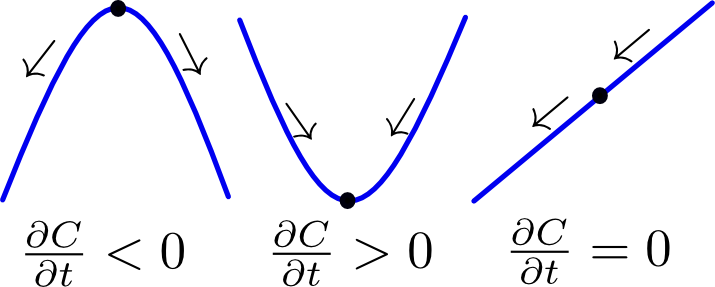#### Figure 1 Illustration of the heat equation. Click to enlarge.

In the videoplayer below we demonstrate how the heat equation smoothes out an initially heterogeneous concentration profile. When only diffusion is acting on the system, it will always evolve to a uniformly flat concentration profile, regardless of the initial conditions.

## Agents

To model bacteria movement on the other hand, we used an agent-based model that explicitly stored individual bacteria as agents. Spatial coordinates are associated with each agent, specifying their location. After solving the equation of motion for all agents based on their environment, these coordinates are updated at every timestep. In principle, Newton’s second law of motion has to be solved for all bacteria. However, since bacteria live in a low Reynolds (high friction) environment, the inertia of the bacteria can be neglected. This is because an applied force will immediately be balanced out by an opposing frictional force, with no noticeable acceleration or deceleration phase taking place. This eliminates the inertial term and simplifies Newton’s second law to (5).

$$\frac{d^2 \vec{r}(t)}{dt^2}=\sum_{i} \vec{F}_{applied,i}-\gamma \cdot \frac{d \vec{r}(t)}{dt}=0$$ $$\Rightarrow \frac{d \vec{r}(t)}{dt}=\frac{1}{\gamma} \cdot \sum_{i} \vec{F}_{applied,i} \;\;\; \text{(5)}$$

Basically, the velocity can be calculated as the sum of all applied forces times divided by a frictional coefficient. For more info about the Reynolds number and “life at low Reynolds number”, we refer to box 2. In the following paragraphs we will investigate the different forces acting on the bacteria and ultimately superimpose them to obtain the final equation of motion.

## Life at Low Reynolds Number

The Reynolds number in fluid mechanics is a dimensionless number that characterizes different flow regimes. It is most commonly used to determine whether laminar or turbulent flow will take place in a hydrodynamic system (Figure 2).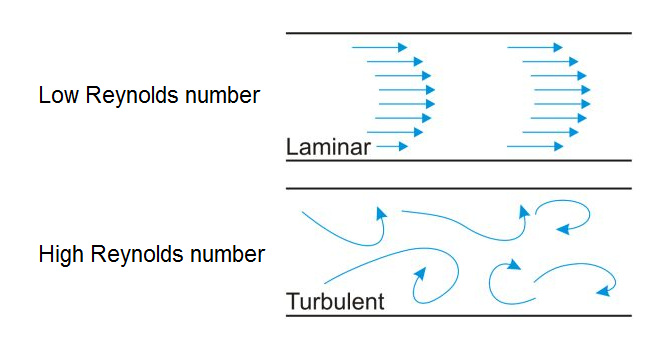#### Figure 2 Flow regimes for different Reynolds numbers. Click to enlarge.

In general however, it quantifies the ratio of inertial forces to viscous forces and is defined as (B2.1).

$$Re=\frac{\text{inertial forces}}{\text{viscous forces}} =\frac{\rho v^2 L^2}{\eta v L} =\frac{\rho v L}{\eta} \;\;\; \text{(B2.1)}$$

For example, if the Reynolds number is high, the inertia of fluids in motion dominate and turbulent flow will occur. When it is low however, the viscous forces dampen the kinetic energy of fluid particles and stabilize the flow profile, ultimately achieving a regular, laminar flow.

The reason we mention the Reynolds number however is not to study the flow of fluids, but to characterize the behavior of swimming objects inside of a stationary fluid. When applied to objects in a fluid, the Reynolds number tells us whether their inertia can be neglected or not. For bacteria, the characteristic length $L$ is on the order of micrometers, which is quite small. Therefore the Reynolds number is always very small and hence viscous forces dominate the motion of bacteria. This is what allows us to eliminate the inertial term in Newton's second law and greatly simplify the equation of motion.

To justify this simplification we will go through a numerical example. Take a bacterium of size $L = 2 a = 1 \cdot \mu m$, swimming through water with a speed of $v=20 \cdot \mu m/s$. Water has a density of $\rho = 1 \; 000 \cdot kg/m^3$ and a viscosity of $\eta = 0.001 \cdot Pa/s$.

Putting these values in (B2.1) yields a Reynolds number of $Re = 2 \cdot 10^{-5} << 1$. Clearly we are in an extremely low Reynolds number regime. To show what this really means, suppose the bacterium stops propelling itself. How long will it continue to move relying only on its inertia? Assuming Stokes' law, we obtain (B2.2) as the equation of motion.

$$F=m\cdot \frac{d^2x}{dt^2} \;\;\; \text{(B2.2a)}$$ $$-6 \pi \eta a v=m\cdot \frac{dv}{dt} \;\;\; \text{(B2.2b)}$$

Solving this differential equation yields (B2.3).

$$v=v_0 \cdot \text{exp} \Bigg[ -\frac{6 \pi \eta a}{m} \cdot (t-t_0) \Bigg] \;\;\; \text{(B2.3)}$$

The characteristic time constant is $\tau = 6\pi\eta a/m \approx 0.1 \cdot \mu s$, from which we calculate that the total distance traversed is $\Delta x < 2 \cdot pm$. This coasting distance is 6 orders of magnitude smaller than its size. Moreover, the time spent coasting is extremely short. Thus, once the bacterium stops propelling itself, it is safe to assume that whatever kinetic energy it had is immediately absorbed by hydrodynamic friction, instantly halting the bacterium. Therefore, we can neglect the inertial term in Newton's second law (5).

## Stochastic Differential Equation

When we try to calculate the physical “chemotactic force”, propelling bacteria towards chemoattractants or away from chemorepellents, we find that it is not easily measured nor derived. Therefore, as a workaround we base the equation of motion on (6), a stochastic differential equation (SDE) that describes the motion of a single particle in a N-particle system that is governed by a Keller-Segel type PDE in the limit of $N \rightarrow \infty$. This Keller-Segel type PDE (7) describes the evolution of a bacteria density field $n$ moving towards some chemoattractant $S$.

$$d\vec{r}_i(t)=\chi (S) \cdot \nabla S(\vec{r},t)\cdot dt + \sqrt{2 \cdot \mu}\cdot d\vec{W} \;\;\; \text{(6a)}$$ $$d\vec{r}_i(t)=\mu \cdot \frac{\kappa}{S(\vec{r},t)} \cdot \nabla S(\vec{r},t)\cdot dt + \sqrt{2 \cdot \mu}\cdot d\vec{W} \;\;\; \text{(6b)}$$

$$\frac{\partial n(\vec{r},t)}{\partial t}=\mu \cdot \nabla^2 n - \nabla (n \cdot \chi(S) \cdot\nabla S(\vec{r},t)) \;\;\; \text{(7a)}$$ $$\frac{\partial n(\vec{r},t)}{\partial t}=\mu \cdot \nabla^2 n - \nabla (n \cdot \mu \cdot \frac{\kappa}{S(\vec{r},t)} \cdot\nabla S(\vec{r},t)) \;\;\; \text{(7b)}$$

Put differently, when infinitely many particles obey (6), they will exhibit Keller-Segel type spatial dynamics as in (7). In a sense, we’re using a “reverse-engineered” particle equation that corresponds to the Keller-Segel field equation. A detailed theoretical treatment of (6) is outside the scope of this model description because it contains a stochastic variable. The traditional rules of calculus do not apply anymore for stochastic differential equations and a different mathematical theory called Itô calculus is required. It is sufficient to say that the second term containing $dW$ accounts for Brownian motion in the form of random noise added to the displacement of the agents, causing them to diffuse, and that it is governed by the diffusion coefficient $\mu$.

The first term in (6) on the other hand is easily understood as an advective or drift term (net motion) dependent on S, pushing the agents along a positive gradient (for negative chemotaxis the sign is reversed). The chemotactic drift hence points towards an increasing concentration of the chemoattractant. The advective properties are governed by the chemotactic sensitivity function $\chi (S)$. For our model we used a function of the form (8).

$$\chi(S)=\mu \cdot \frac{\kappa}{S(\vec{r},t)} \;\;\; \text{(8)}$$

The first important thing to note is that we assume $\chi (S)$ to be proportional to $1/S$. This is because Keller and Segel proved that their corresponding PDE model only yields travelling wave solutions if $\chi (S)$ contains such a singularity at some critical concentration $S_{crit}$, and multiplying by $1/S$ is the simplest way to introduce a singularity at $S_{crit} = 0$. Secondly, the proportionality constant is composed of two factors, namely the bacterial diffusion coefficient $\mu$ and chemotactic sensitivity constant $\kappa$. This is done for two reasons. Firstly, when $\mu$ is lowered, both chemotactic and random motion is reduced, which emulates the state of inactivated motility due to high or low concentrations of AHL. Secondly, defining a separate chemotactic sensitivity constant allows us to examine the effect of the relative strength of chemotaxis versus random motion on pattern formation. Conceptually, this term can be viewed as a sort of chemotactic force, defined with regards to a mobility coefficient $\mu$ instead of a frictional coeffient, which are the inverse of each other.

## Cell-Cell Interactions

In addition to chemotaxis and diffusion, cell-cell interactions play an important role in pattern formation and also need to be modeled. Bacteria have finite size and therefore multiple bacteria cannot occupy the same space. Moreover, an important mechanism in our system is the aggregation of cells A due to the sticky adhesin protein membrane. To take these mechanisms into account we modeled two types of cell-cell interactions: the purely repulsive interaction of cell B with another cell B and with cell A, and the attractive-repulsive interaction of cell A with another cell A. The interaction between two cells is usually expressed by a potential energy curve defined over the distance between the centers of mass of the two cells (Figure 4).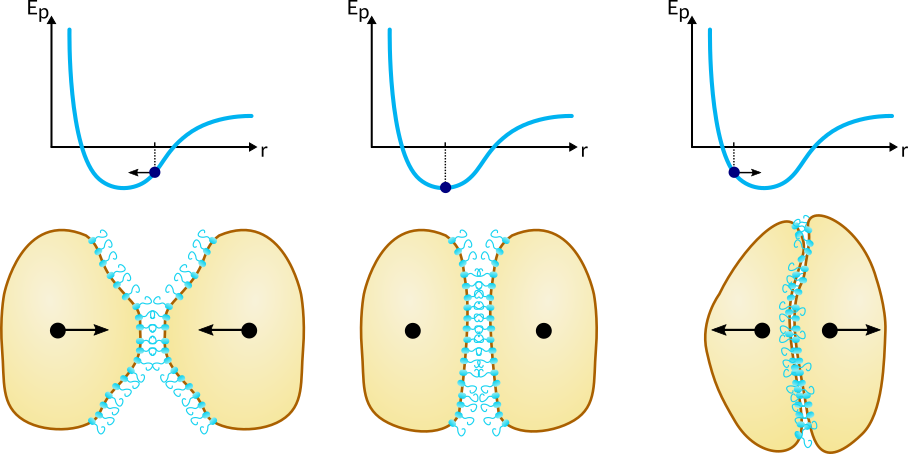#### Figure 4Cell-cell interaction potential. Click to enlarge.

Note that the potential energy remains constant after a certain distance, which means that the cells stop interacting. Also, as two cells move closer together, they hit a wall where the potential energy curve abruptly goes to infinity. The reason for this is that two cells cannot occupy the same space and therefore smaller intercellular distances are not allowed. Implementing this theoretical potential is however not possible because the displacement of bacteria is a stochastic process and the bacteria could randomly jump beyond the potential wall, where the force is ill defined. Therefore, we’ve decided to define a piecewise quadratic potential (9), which results in a piecewise linear force that resembles Hooke’s law, but with three different “spring constants” acting in different intervals of intercellular distances (10), as illustrated in Figure 5. The force is defined with respect to the unit vector pointing towards the other cell, meaning that a positive force corresponds to an attractive force and vice-versa.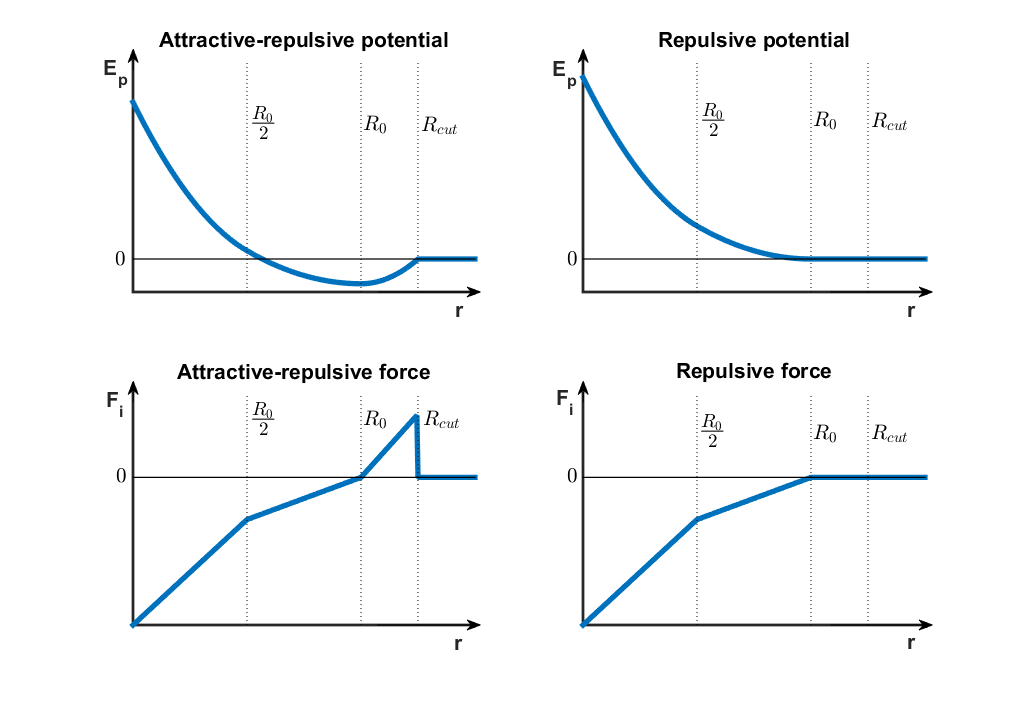#### Figure 5Cell-cell interaction potential and force curves. Click to enlarge.

$$E_{p,attr-rep}(r_{ij})=\left\{\begin{matrix} 0 & R_{cutoff}\leq r_{ij}\\ \frac{1}{2}\cdot k_3 \cdot(r_{ij}-R_0)^2+C_1 & R_0 \leq r_{ij} < R_{cutoff} \\ \frac{1}{2}\cdot k_2 \cdot(r_{ij}-R_0)^2+C_1 & \frac{R_0}{2} \leq r_{ij} < R_0\\ \frac{1}{2}\cdot k_1 \cdot(r_{ij}-\frac{k_1+k_2}{k_1}\cdot \frac{R_0}{2})^2+C_2 & 0 \leq r_{ij} < \frac{R_0}{2} \end{matrix}\right. \;\;\; \text{(9a)}$$ $$E_{p,rep}(r_{ij})=\left\{\begin{matrix} 0 & R_0\leq r_{ij}\\ \frac{1}{2}\cdot k_2 \cdot(r_{ij}-R_0)^2 & \frac{R_0}{2} \leq r_{ij} < R_0\\ \frac{1}{2}\cdot k_1 \cdot(r_{ij}-\frac{k_1+k_2}{k_1}\cdot \frac{R_0}{2})^2 & 0 \leq r_{ij} < \frac{R_0}{2} \end{matrix}\right. \;\;\; \text{(9b)}$$

$$\vec{F}_{i,attr-rep}(r_{ij})= \frac{\partial E_{p,attr-rep}(r_{ij})}{\partial r_{ij}} \cdot \vec{e}_{ij}= \left\{\begin{matrix} \vec{0} & R_{cutoff}\leq r_{ij}\\ k_3 \cdot(r_{ij}-R_0) \cdot \vec{e}_{ij} & R_0 \leq r_{ij} < R_{cutoff} \\ k_2 \cdot(r_{ij}-R_0) \cdot \vec{e}_{ij} &\frac{R_0}{2} \leq r_{ij} < R_0\\ k_1 \cdot(r_{ij}-\frac{k_1+k_2}{k_1}\cdot \frac{R_0}{2}) \cdot \vec{e}_{ij} & 0 \leq r_{ij} < \frac{R_0}{2} \end{matrix}\right. \;\;\; \text{(10a)}$$ $$\vec{F}_{i,rep}(r_{ij})= \frac{\partial E_{p,rep}(r_{ij})}{\partial r_{ij}} \cdot \vec{e}_{ij}= \left\{\begin{matrix} \vec{0} & R_0\leq r_{ij}\\ k_2 \cdot(r_{ij}-R_0) \cdot \vec{e}_{ij} & \frac{R_0}{2} \leq r_{ij} < R_0\\ k_1 \cdot(r_{ij}-\frac{k_1+k_2}{k_1}\cdot \frac{R_0}{2}) \cdot \vec{e}_{ij} & 0 \leq r_{ij} < \frac{R_0}{2} \end{matrix}\right. \;\;\; \text{(10b)}$$

Between A type cells, there is a region of attraction $(R_0 \leq r_{ij} < R_{cutoff})$, where the force points towards the other cell, hence moving them closer together. In the repulsive domain $(r_{ij} < R_0)$, two regions were defined, emulating lower repulsive forces $(\frac{R_0}{2} \leq r_{ij} < R_0)$ and higher repulsive forces due to a higher spring constant when the cells are even closer $(r_{ij} < \frac{R_0}{2})$. For the purely repulsive interaction scheme there is no attraction and therefore the spring constant for $R_0 \leq r_{ij}$ is zero. More details about the implementation of the cell-cell interaction scheme, more specifically regarding the nearest-neighbor search algorithm, can be found in the paragraph on the agent-based module below.

## Equation of Motion

Now we are ready to construct the equation of motion for cell type A and B as a superposition of an adapted Keller-Segel SDE (6) and cell-cell interaction forces (10), yielding (11).

$$d\vec{r}_{A_i}(t)= \sqrt{2 \cdot \mu_A (H)}\cdot d\vec{W} + \frac{1}{\gamma}\cdot\Bigg[ \sum^{A \backslash \{ A_i\}}_j \frac{dE_{p,attr-rep}(r_{ij})}{dr_{ij}} \cdot \vec{e}_{ij} +\sum^{B}_j\frac{dE_{p,rep}(r_{ij})}{dr_{ij}}\cdot \vec{e}_{ij} \Bigg]\cdot dt \;\;\; \text{(11a)}$$ $$d\vec{r}_{B_i}(t)= \sqrt{2 \cdot \mu_B(H)}\cdot d\vec{W} + \chi(L,H) \cdot \nabla L(\vec{r},t)\cdot dt + \frac{1}{\gamma}\cdot\Bigg[ \sum^{A\cup B\backslash \{ B_i\}}_j \frac{dE_{p,rep}(r_{ij})}{dr_{ij}}\cdot \vec{e}_{ij} \Bigg]\cdot dt \;\;\; \text{(11b)}$$

$$\chi(L,H)= -\mu_{B}(H) \cdot \frac{\kappa}{L(\vec{r},t)} \;\;\; \text{(12)}$$

$$\mu_A(H)=\left\{\begin{matrix} \mu_{A,high} & H(\vec{r},t) < H_{A,threshold}\\ \mu_{A,low} & H(\vec{r},t) \geq H_{A,threshold} \end{matrix}\right. \;\;\; \text{(13a)}$$ $$\mu_B(H)=\left\{\begin{matrix} \mu_{B,high} & H(\vec{r},t) < H_{B,threshold}\\ \mu_{B,low} & H(\vec{r},t) \geq H_{B,threshold} \end{matrix}\right. \;\;\; \text{(13b)}$$

Bacteria of type A are not attracted nor repelled by leucine, so the chemotactic term falls away. All cell-cell forces are summed up to find a net force, taking into account the two different potentials due to the different interaction types. As discussed before, this net force times a constant yields the velocity due to that force, which is then multiplied by $dt$ to obtain the displacement. For B type cells, the chemotactic term models the repulsive chemotaxis away from leucine. The chemotactic sensitivity function (12) has a negative sign signifying that B type cells are repelled by leucine. The cell-cell interaction term in this case is simpler because B type cells only interact repulsively.

Note that the diffusion coefficient of cell types A and B (13) switches based on the local concentration of AHL relative to a threshold AHL value, which simulates the dependency of cellular motility on AHL. For B type cells the cellular motility depends explicitly on AHL due to the synthetic genetic circuit we have built into them. On the other hand, in our model the motility of A type cells should not depend on AHL directly, but since high concentrations of AHL are caused by high densities of aggregated A type cells, the bacteria typically will not be motile in high AHL concentrations because they stick to neighboring cells.

The agent-based module is now fully defined but one crucial issue was skipped: AHL and leucine concentrations are calculated using PDEs and are therefore only known at grid points. Agents on the other hand reside in the space between grid points and require local concentrations as inputs to calculate their next step. This problem is part of the coupling aspect in our hybrid modeling framework and is discussed below.

## Coupling

At this point, both the PDE module and agent-based module have been established, but the issue remains that the individual modules take inputs and return outputs which are not directly compatible. In the framework of PDEs, entities are described by density/concentration fields. In the agent-based module however, entities are represented by discrete objects with exact spatial locations. The key challenge posed by the hybrid model is to integrate these different approaches by bidirectionally converting and exchanging information between the modules. Coupling the modules is the essential component that makes the hybrid model hybrid. It allows us to leverage the advantages of both modeling approaches, while circumventing their drawbacks. But although hybrid modeling opens up many new avenues for novel modeling methods, it comes with its own diverse set of issues and peculiarities that need to be addressed before it can be successfully applied.

In the following paragraphs the basic scheme for coupling the PDE and agent-based modules in our model is introduced, after which the theoretical treatment of our hybrid model is complete. The difficulties that arise from linking the modules together are discussed further below in the subsection on matching.

## Agent-Based to PDE

As described above, the agents’ effect in the PDE is modeled as a source term that is proportional to the agent density. This approach is essentially the same approach taken in the colony level model for the bacterial production of AHL and leucine. However, in the colony level model the bacteria density is explicitly calculated at the grid points, while the agent-based model essentially considers a set of points scattered in space. A simple first-order approach would be to determine the closest grid point to any agent and simply increment a counter belonging to that grid point. This results in a histogram, which can be used directly to represent the agent density. However, the resulting density is a blocky, nonsmooth function which poorly represents the underlying agent distribution. The effect of a single agent is artificially confined to a single grid point, while in reality an agent’s influence could reach much further than a single grid point. The shape of a histogram is also very dependent on the bin size, which in this case corresponds to the grid spacing so it cannot be independently tuned. To decouple grid spacing and agent density and achieve a smoother density function, we made use of a more sophisticated technique called kernel density estimation (KDE).

KDE is used in statistics to estimate the probability density of a set discrete data derived from a random process. The basic idea consists of defining a kernel function that represents the density of a single data point, then centering kernel functions on every data point and summing them all up to achieve a smooth overall density function, as demonstrated in the Figure 6.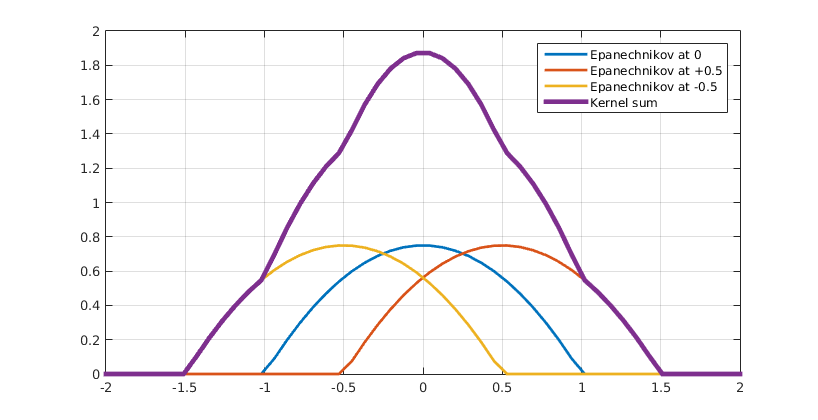#### Figure 6 Kernel density estimation. Click to enlarge.

This kernel function can be anything as long is it continuous, symmetric and integrates to 1, since it represents one data point or one agent in our case. Some of the most common kernel functions include Gaussian kernels, triangular kernels and Epanechnikov kernels (14), which are shown in Figure 5.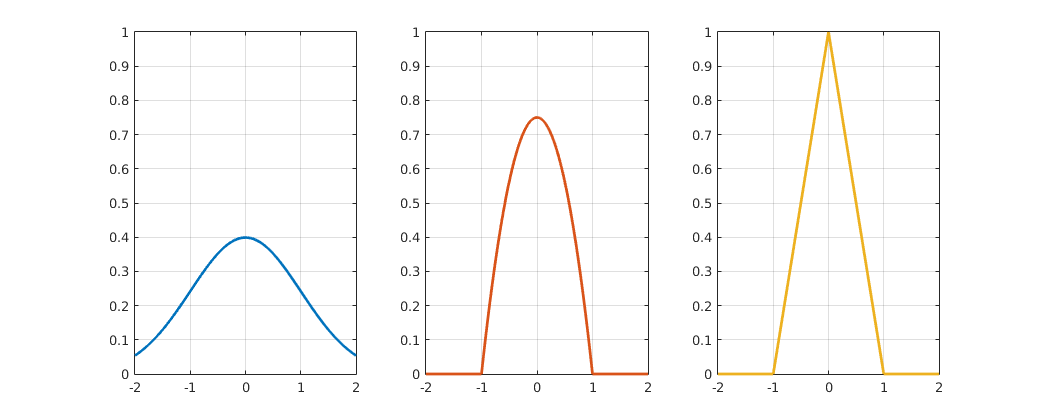#### Figure 7 Gaussian, triangular and Epanechnikov kernel functions. Click to enlarge.

$$K_{Gaus}(x)=\frac{1}{\sqrt{2 \cdot \pi}} \cdot e^{-\frac{1}{2}x^2} \;\;\; \text{(14a)}$$ $$K_{tri}(x)=\left\{\begin{matrix} 0 & 1 < |x| \\ 1-|x| & |x| \leq 1 \end{matrix}\right. \;\;\; \text{(14b)}$$ $$K_{Epa}(x)=\left\{\begin{matrix} 0 & 1 < |x| \\ \frac{3}{4} \cdot (1-x^2) & |x| \leq 1 \end{matrix}\right. \;\;\; \text{(14c)}$$

In practice, scaled versions of standard kernel functions are used, which are of the form (15).

$$K_h(x)=\frac{1}{h} \cdot K \bigg( \frac{x}{h} \bigg) \;\;\; \text{(15)}$$

Importantly, the scaled functions inherit the kernel function properties, but are either broader or narrower. The degree to which the shape of a kernel function is stretched or squeezed depends on the scaling factor h, which is why it is called the bandwidth, see Figure 6.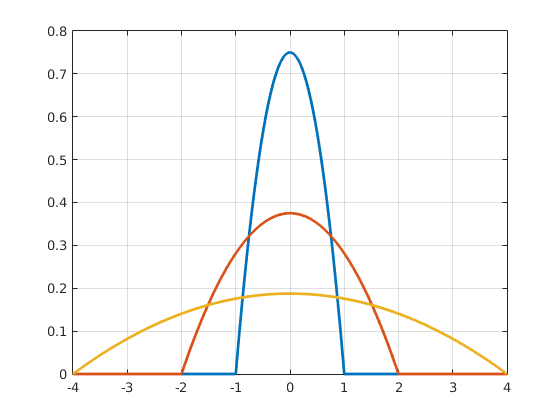#### Figure 8Epanechnikov kernels using different bandwidths. Click to enlarge.

This parameter gives us the freedom to define how far the influence of an agent reaches and how smooth the resulting density function looks like. An example is given in Figure 7, where 100 agents were sampled from a normal distribution. The red dotted line indicates the true underlying distribution, while the blue line is the kernel density estimation calculated using different bandwidths. The left graph shows a undersmoothed (small bandwidth) KDE, which oscillates wildly. The right graph on the other hand is oversmoothed (large bandwidth), leading to a misleadingly wide KDE.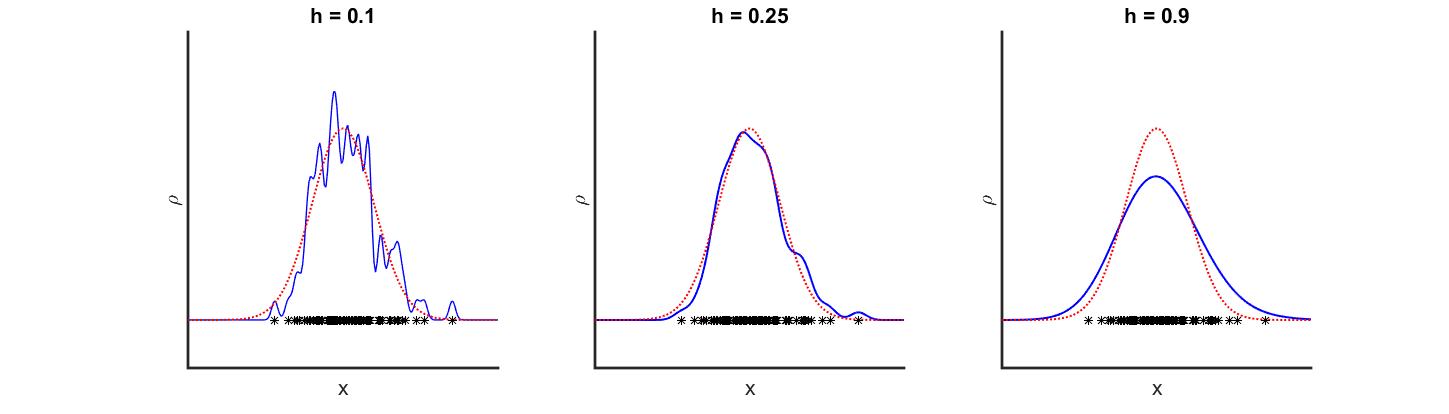#### Figure 9Kernel density estimation using different bandwidths. Click to enlarge.

In summary, using kernel density estimation we can express the agent density in the form of (16), providing the appropriate input for the PDE model.

$$\rho(x)=\beta \cdot \sum^N_{i} K_h(x-x_i) \;\;\; \text{(16)}$$

The extension to higher dimensions is called multivariate kernel density estimation and is rather non-trivial since the bandwidth is then defined as a matrix instead of a scalar, which allows for many more variations on the same basis kernel function. A detailed analysis of multivariate kernel density estimation will not be given here. It suffices to say that for our 2-D hybrid model we used the simplest possible bandwidth matrix, which is the identity matrix times a constant.

## PDE to Agent-Based

The final component of our hybrid model is the mapping of the PDE model to the agent-based model. The latter model works with discrete objects that have continuous coordinates, meaning that they can be located at any point of the domain. As we have seen in (11), the agents need the local concentration of AHL and leucine, as well as the gradient of leucine in order to update their positions. In the PDE model however, the domain is discretized into a grid and concentrations are only defined at grid points. Therefore, in order to transfer information from the PDE model to the agent-based model we need to translate these grid values into values for any given position within the domain. We achieved this for the 1-D hybrid model by taking the two nearest grid points to any agent and employing linear interpolation to derive an approximate local field value. Similarly, for the 2-D hybrid model we took the four nearest grid points and employed bilinear interpolation, as explained in box 3. With the end result (B3.3), the concentration or gradient of AHL or leucine can be derived at any point of the domain. Therefore, the agent-based module has access to the information that it needs from the PDE module.

## Bilinear Interpolation

Interpolation is used to obtain a continuous function when a finite amount of data points are known. Piecewise linear interpolation is the most simple scheme to interpolate 1-dimensional data.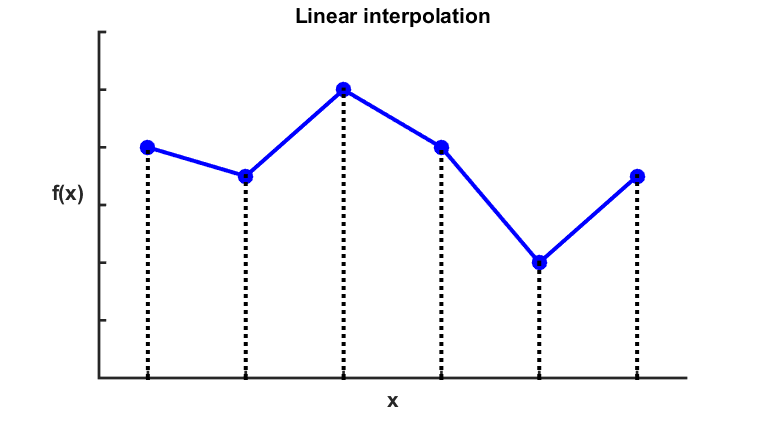#### Figure 10 Illustration of linear interpolation. Click to enlarge.

The technique involves defining linear functions connecting consecutive data points to fill in the gaps, using (B3.1).

$$\hat{f}(x)=f(x_i)+\frac{f(x_{i+1})-f(x_i)}{x_{i+1}-x_i} \cdot (x-x_i) \; \text{for} \; x_i < x < x_{i+1} \;\;\; \text{(B3.1)}$$

Bilinear interpolation is the generalization of this interpolation technique to two dimensions, illustrated using Figure 9.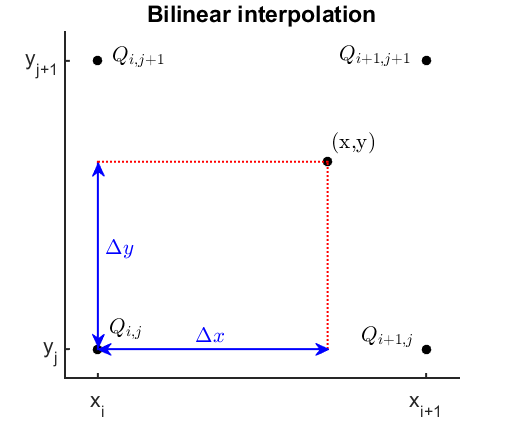#### Figure 11 Illustration of bilinear interpolation. Click to enlarge.

Let’s assume we want to interpolate a two-dimensional function within a rectangular domain where the function values are only known on the four adjacent grid points $Q$. First we linearly interpolate in the x-direction on the lines connecting $Q_{i,j}$ and $Q_{i+1,j}$, $Q_{i,j+1}$ and $Q_{i+1,j+1}$, which yields (B3.2).

$$\hat{f}(x,y_j)=\frac{x_{i+1}-x}{x_{i+1}-x_i} \cdot f(Q_{i,j}) + \frac{x-x_i}{x_{i+1}-x_i} \cdot f(Q_{i+1,j}) \;\;\; \text{(B3.2a)}$$ $$\hat{f}(x,y_{j+1})=\frac{x_{i+1}-x}{x_{i+1}-x_i} \cdot f(Q_{i,j+1}) + \frac{x-x_i}{x_{i+1}-x_i} \cdot f(Q_{i+1,j+1}) \;\;\; \text{(B3.2b)}$$

We then obtain interpolated values for all positions on the bottom and top edge. The key idea then is to take these values and interpolate in the y-direction for any given x-position, yielding (B3.3).

$$\hat{f}(x,y)=\frac{y_{j+1}-y}{y_{j+1}-y_j} \cdot \hat{f}(x,y_j) + \frac{y-y_j}{y_{j+1}-y_j} \cdot \hat{f}(x,y_{j+1}) \\ =\frac{y_{j+1}-y}{y_{j+1}-y_j} \cdot \Bigg[ \frac{x_{i+1}-x}{x_{i+1}-x_i} \cdot f(Q_{i,j}) + \frac{x-x_i}{x_{i+1}-x_i} \cdot f(Q_{i+1,j}) \Bigg] \\ +\frac{y-y_j}{y_{j+1}-y_j} \cdot \Bigg[ \frac{x_{i+1}-x}{x_{i+1}-x_i} \cdot f(Q_{i,j+1}) + \frac{x-x_i}{x_{i+1}-x_i} \cdot f(Q_{i+1,j+1}) \Bigg] \;\;\; \text{(B3.3)}$$

This then gives interpolated values for any point within the domain. Note that the resulting function is not linear, but quadratic since it contains the term $x \cdot y$.

## Synthesis

The diffusion, production and degradation of AHL and leucine are described by the PDEs (4). At the same time, the random movement, chemotaxis and intercellular interactions of cell types A and B are captured by the stochastic equation of motion (11). Translating the spatial distribution of cells type A into a density field to feed into the PDE module can be accomplished by using kernel density estimation (16). Finally, the agents can request concentrations and gradients at arbitrary positions from the PDE module using bilinear interpolation (B3.3). Taken together, these equations describe the individual modules, as well as the two-way information transfer in between them, and thus they fully define our hybrid model. A graphical summary is shown in Figure 10.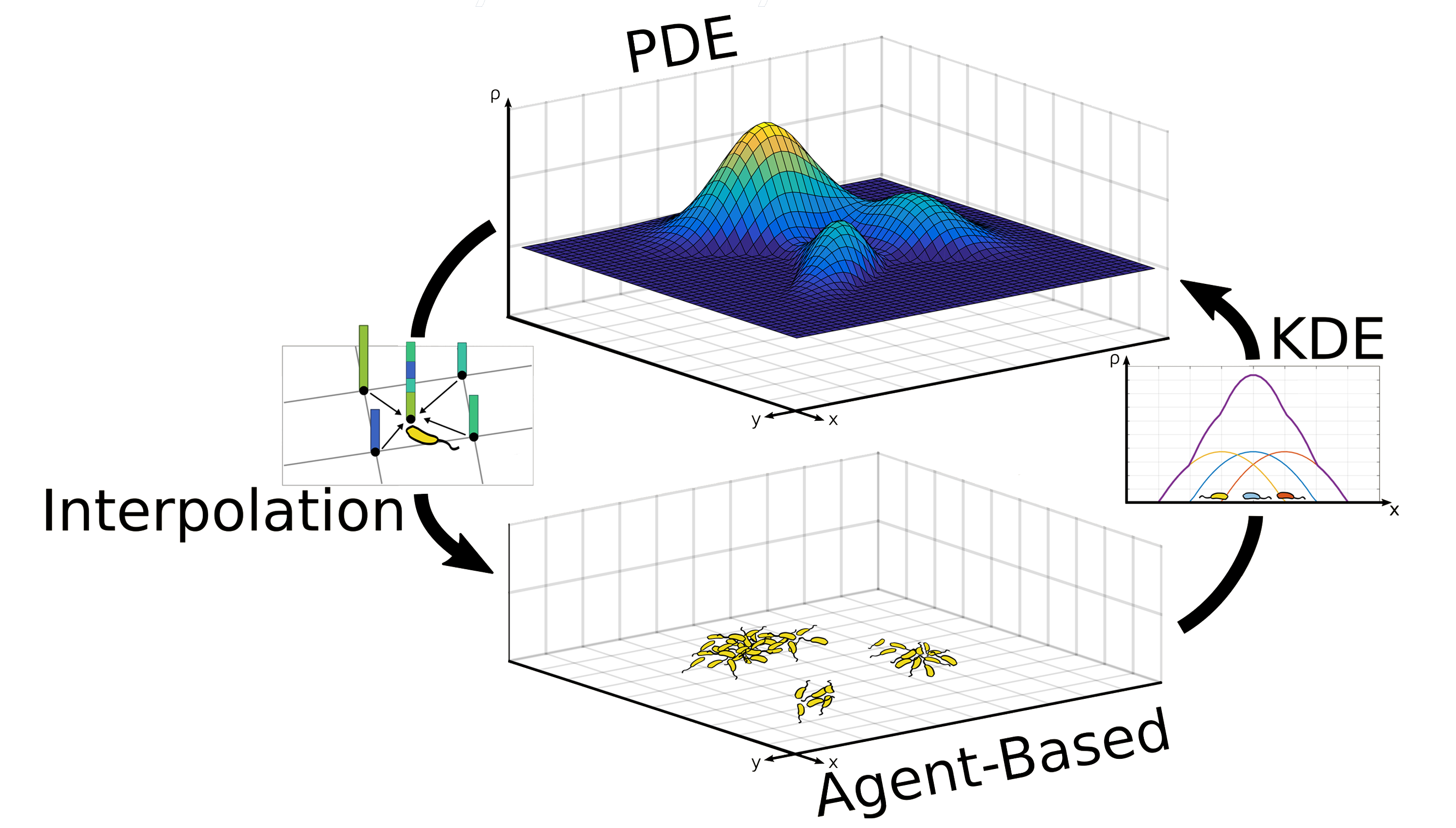#### Figure 12 Graphical summary of hybrid model. Click to enlarge.## Agent-Based Module

In this paragraph, we will discuss the implementation of the agent-based module. First, the problem of computing pairwise interactions is discussed and a nearest-neighbor algorithm is introduced to reduce the computation time. Then, the choice of timestep is considered with regards to the cell-cell interaction scheme. Finally, the boundary conditions for agents are defined and explained.

## Nearest-Neighbor Algorithm

The cell-cell interactions have already been fully described in the paragraphs above. However, solving the equation of motion of an agent in its current form (11) requires the computation of the force due to every other agent. If we take N to be the number of agents, that means $N*(N-1)$ amount of force computations are executed in total and therefore the computation time grows as $O(N^2)$. To put this in perspective, if we simulate a thousand agents, the amount of interactions adds up to one million. This puts a heavy computational load on the model which can be reduced greatly by using better algorithms. The crucial observation here is that the force is zero when the distance between two cells is larger than $2\cdot r_{cutoff}$. An agent therefore only actually interacts with its nearest neighbors. Hence, the naive implementation wastes a lot of time computing interactions which have no effect.

To speed up calculation of cell interactions we used an algorithm that searches only for neighbors within the cutoff distance for every agent. Moreover, since we take the timestep small enough so that agents do not easily move out of each other's influence from one moment to another (see paragraph below), it is not necessary to recompute the neighbors at every timestep. Instead, we maintain a neighbor list for every agent which is refreshed only every few timesteps.

There exist many different algorithms for searching nearest neighbors and the optimal choice of algorithm depends on the characteristics of the spatial distribution of agents. Since in our model cells repel each other when they approach each other too closely, they will evolve to a rather uniform distribution. The most appropriate fixed-radius nearest neighbor search algorithm in that case is the cell technique. In this algorithm, the agents are structured by placing a rectangular grid of cells over the domain and assigning every agent to a cell. Determining which cell an agent belongs to is easily done by rounding the x and y-coordinates down to the nearest multiple of the grid spacing. In Figure 10 agent 1 and agent 2 have different coordinates but $x \; mod \; d = x_k$ and $y \; mod \; d = y_k$ for both agents and therefore they will be assigned to the same cell k.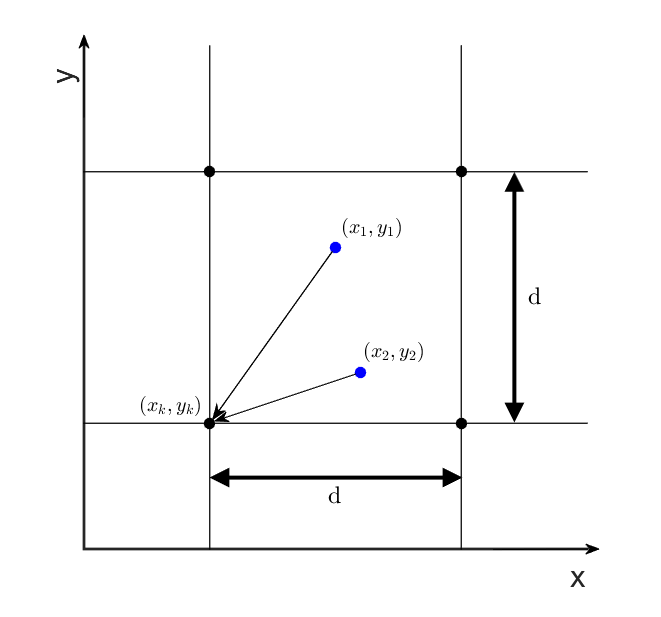#### Figure 13 Cell assignment. Click to enlarge.

If the grid spacing is chosen so that interactions do not reach further than the adjacent cells, every agent only needs to look for neighbors in 9 cells (its own cell plus 8 adjacent cells) instead of the entire domain, as illustrated in Figure 12.## Choice of Timestep

In contrast to PDE models, there is no danger of instability due to a large timestep in agent-based models. However, since the time step determines how far a cell can “jump” away from its previous position, we need to ensure that it is not so large as to negate the effect of intercellular interactions. To understand this, we first discretize the Brownian motion term in the agent’s equation of motion (17).

$$\sqrt{2 \cdot \mu}\cdot d\vec{W} \rightarrow \sqrt{2 \cdot \mu \cdot \Delta t}\cdot \vec{N}(0;1) \;\;\; \text{(17)}$$

The details of this discretization scheme goes beyond the scope of this text and will not be given here. It can be proven that this term follows a distribution as (18).

$$\sqrt{2 \cdot \mu \cdot \Delta t}\cdot \vec{N}(0;1) \sim \vec{N}(0;2 \cdot \mu \cdot \Delta t) \;\;\; \text{(18)}$$

Now assume that there are two A type cells who are exactly $2 \cdot r_0$ apart. If the timestep is too large, they might “jump” out of each other’s influence at the next iteration. This situation is highly unrealistic because the cells actually should feel each other’s pull while they randomly drift away from each other. If the timestep is too large, there’s a high probability that the cells detach without experiencing the potential at all and therefore the probability of detachment is artificially inflated. Of course, we can never completely eliminate the possibility of a sudden detachment because the random is sampled by a normal distribution, but we can define a desired probability of sudden detachment $P^-$. Then, for a given $r_0$, $r_{cutoff}$ and $\mu$, we can determine how small the timestep has to be for the sudden detachment to happen only with probability $P^-$. First, we assume two A type cells with coordinates $(x_1,y_1)$ and $(x_2,y_2)$ that are at a distance $2 \cdot r_0 = R_0$ from each other. We then define the coordinates at the next timestep as stochastic variables $X_1$, $Y_1$, $X_2$ and $Y_2$, which are distributed as (19).

$$X_1 \sim x_1 + \sqrt{2 \cdot \mu \cdot \Delta t}\cdot {N}(0;1) \sim {N}(x_1;2 \cdot \mu \cdot \Delta t) \;\;\; \text{(19a)}$$ $$Y_1 \sim y_1 + \sqrt{2 \cdot \mu \cdot \Delta t}\cdot {N}(0;1) \sim {N}(y_1;2 \cdot \mu \cdot \Delta t) \;\;\; \text{(19b)}$$ $$X_2 \sim x_2 + \sqrt{2 \cdot \mu \cdot \Delta t}\cdot {N}(0;1) \sim {N}(x_2;2 \cdot \mu \cdot \Delta t) \;\;\; \text{(19c)}$$ $$Y_2 \sim y_2 + \sqrt{2 \cdot \mu \cdot \Delta t}\cdot {N}(0;1) \sim {N}(y_2;2 \cdot \mu \cdot \Delta t) \;\;\; \text{(19d)}$$

We are not interested in the future coordinates as such, but rather in the future intercellular distance, which is composed of these future coordinates and therefore also is a stochastic variable. For ease of calculation, we work with the square of this variable, which we name as R² and define as (20).

$$R^2=(X_1-X_2)^2 + (Y_1-Y_2)^2 \;\;\; \text{(20)}$$

It is easy to see that $X_1-X_2$ and $Y_1-Y_2$ are both noncentral (mean is not equal to zero) normally distributed variables as well. Therefore $R^2$ obeys a noncentral chi-squared distribution with 2 degrees of freedom. Before proceeding further, we have to make our $R^2$ conform to a standard noncentral chi-squared distribution. First we normalize $X_1-X_2$ and $Y_1-Y_2$. We observe that $X_1-X_2$ and $Y_1-Y_2$ are distributed as (21).

$$\begin{split} X_1-X_2 & \sim N(x_1-x_2; 2 \cdot \mu \cdot \Delta t + 2 \cdot \mu \cdot \Delta t) \\ & \sim N(x_1-x_2; 4 \cdot \mu \cdot \Delta t) & \;\;\; \text{(21a)} \end{split}$$ $$\begin{split} Y_1-Y_2 & \sim N(y_1-y_2; 2 \cdot \mu \cdot \Delta t + 2 \cdot \mu \cdot \Delta t) \\ & \sim N(y_1-y_2; 4 \cdot \mu \cdot \Delta t) & \;\;\; \text{(21b)} \end{split}$$

Secondly, because the means of the $X_1-X_2$ and $Y_1-Y_2$ are not equal to zero, we need to account for this by calculating the noncentrality parameter $\lambda$. This is equal to the sum of squares of the means of the normalized $X_1-X_2$ and $Y_1-Y_2$ variables, which of course equals (22), corresponding to a scaled version of the original intercellular distance.

$$\begin{split} \lambda & = \Bigg(\frac{x_1-x_2} {2 \cdot \sqrt{ \mu \cdot \Delta t}}\Bigg)^2 + \Bigg(\frac{y_1-y_2} {2 \cdot \sqrt{ \mu \cdot \Delta t}}\Bigg)^2 \\ & = \frac{(x_1-x_2)^2+(y_1-y_2)^2} {4 \cdot \mu \cdot \Delta t} \\ & = \frac{r^{2}_{0}}{4 \cdot \mu \cdot \Delta t} & \;\;\; \text{(22)} \end{split}$$

We then define the standardized variable $\bar{R}^2$ as (23).

$$\begin{split} \bar{R}^2 & = \Bigg(\frac{X_1-X_2} {2 \cdot \sqrt{ \mu \cdot \Delta t}}\Bigg)^2 + \Bigg(\frac{Y_1-Y_2} {2 \cdot \sqrt{ \mu \cdot \Delta t}}\Bigg)^2 \\ & \sim \chi^2 (2; \frac{r^{2}_{0}}{4 \cdot \mu \cdot \Delta t}) \\ & \sim \chi^2 (2; \lambda) & \;\;\; \text{(23)} \end{split}$$

To recap, the meaning of $\bar{R}^2$ is the scaled squared intercellular distance after updating once with timestep $\Delta t$ starting from an initial distance of $r_0$. Then we define $F$ as the cumulative distribution function for the random variable $\bar{R}^2$ as (24).

$$F(\bar{R^*}^2) = P(\bar{R}^2 \leq \bar{R^*}^2) \;\;\; \text{(24)}$$

To then finally calculate appropriate timestep for a given $r_0$, $r_{cutoff}$, $\mu$ and desired $P^-$ we take the inverse cumulative distribution function and equate it to the intercellular cutoff distance, after appropriate scaling and squaring (25).

$$F^{-1}(1-P^-) = \bar{r}_{cutoff}^{2} = \frac{r_{cutoff}^{2}}{4 \cdot \mu \cdot \Delta t} \;\;\; \text{(25)}$$

Note that both the left hand side and right hand side both depend on the $\Delta t$ in a nonlinear way, therefore a nonlinear solver is required to solve this equation. As stated previously, it is not possible to completely avoid that the cells will jump out of each other's influence in a single timestep. However, equation (25) allows us to calculate the timestep $\Delta t$ that will result in cells detaching in a single timestep with a probability $P^-$ that we can specify.

## Boundary Conditions

Solving the equation of motion to compute trajectories of bacteria is well-defined as long as they stay within in the domain. To give a complete picture however, we also need to specify what agents should do when they attempt to cross the spatial boundaries of the simulation. Initially we implemented a simple “freeze” boundary condition, which means that the bacteria are simply forced back to the edge whenever they tried to exit the domain. However, as our model got more sophisticated it became apparent that simulating an entire petri dish would not be feasible. Therefore, under the assumption that our system would produce similar patterns over the entire petri dish, we decided to implement periodic boundary conditions. This means that we treat the simulated domain as a “unit cell” which is repeated ad infinitum in all spatial directions. As a result, an agent that tries to exit the simulation box at one side re-enters from the opposite side, like in Figure 13.#### Figure 15 Periodic boundary conditions. Click to enlarge.

Periodic boundary conditions are easily implemented by taking the modulus of every coordinate with the length of the periodic domain in that dimension as modulo (26).

$$x' = x \; mod \; L_x \;\;\; \text{(26a)}$$ $$y' = y \; mod \; L_y \;\;\; \text{(26b)}$$

One last complication is that cell-cell interactions across domain edges are possible with periodic boundary conditions. To compute those, virtual cells containing virtual agents are used that represent real cells and agents close to the opposite edge of the domain, but are translated to appear to come from outside the domain.

## Partial Differential Equations Module

To implement the partial differential equations (4) we have to discretize our domain into a grid and apply some computational scheme that describes the evolution of our fields in discrete timesteps. We will discuss only the scheme that we employed for the two-dimensional model. Furthermore, boundary conditions are described below as well.

## Computational Scheme

As mentioned earlier the concentrations of AHL and leucine are modeled using partial differential equations. In the colony level model these equations are solved explicitly. Explicit schemes do not require a lot of work per timestep, but unfortunately are not unconditionally stable. If $\Delta t$ the timestep, $\Delta x$ and $\Delta y$ the distances between grid points in the x and y-direction respectively, and $D$ the diffusion coefficient, the condition for stability is given by (27).

$$D \cdot \Bigg( \frac{\Delta t}{\Delta x^2} + \frac{\Delta t}{\Delta y^2} \Bigg) \leq \frac{1}{2} \;\;\; \text{(27)}$$

When computing the solution of the hybrid model this requirement forces us to spend a lot of CPU time solving partial differential equations that could be better spent simulation the agents. Therefore an implicit alternating direction implicit (ADI) scheme was implemented. ADI schemes are unconditionally stable, which allows us to take large time steps with the PDE solver. The difference from a To derive the scheme we first reiterate the partial differential equation (4).

$$\frac{\partial C(\vec{r},t)}{\partial t}=D \cdot \nabla^2 C(\vec{r},t)+\alpha \cdot \rho_A(\vec{r},t)-k\cdot C(\vec{r},t) \;\;\; \text{(4)}$$

We could use a fully implicit scheme by taking the field values on the right-hand side at $t+\Delta t$, but there is no way to reshape the resulting matrix to take on a banded form and hence no efficient solver is applicable to compute the field values at the next timestep. The trick used in ADI is then to divide the timestep $\Delta t$ in two half timesteps and take only one dimension implicitly at a time, and leave the other dimension in its explicit form. In the subsequent half timestep, the dimensions are switched (hence alternating). For example, if we take $x$ implicitly in the first half, we obtain (28a).

$$\frac{C^{n+1/2}-C^{n}}{1/2 \cdot \Delta t}=D \cdot \Bigg( \frac{\delta^2_x C^{n+1/2}}{\Delta x^2} +\frac{\delta^2_y C^{n}}{\Delta y^2} \Bigg) +\alpha \cdot \rho_A^{\bar{n}} -k\cdot \frac{1}{2} \cdot \big(C^{n+1/2}+C^n \big) \;\;\; \text{(28a)}$$

The density of type A bacteria $\rho_A$ might evolve over multiple timesteps in $[t;t+\Delta t]$ due to decoupling of timesteps (see Matching below), therefore we compute an average over the entire interval and use that in the discretization scheme. Furthermore, the degradation term can be incorporated in a Crank-Nicholson manner since they do not depend on neighboring grid points and therefore do not influence the shape of the matrix. We define $r_x$ and $r_y$ as in (29).

$$r_x = D\cdot \frac{\Delta t}{\Delta x^2} \;\;\; \text{(29a)}$$ $$r_y = D\cdot \frac{\Delta t}{\Delta y^2} \;\;\; \text{(29b)}$$

Inserting these in (28a) and moving all implicit terms to the left-hand side and all explicit terms to the right-hand side gives (28b).

$$\Bigg(1+\frac{1}{4}\cdot k \cdot \Delta t - \frac{1}{2} \cdot r_x \cdot \delta^2_x \Bigg) \cdot C^{n+1/2} = \Bigg(1-\frac{1}{4}\cdot k \cdot \Delta t + \frac{1}{2} \cdot r_y \cdot \delta^2_y \Bigg) \cdot C^{n} + \frac{1}{2} \cdot \alpha \cdot \Delta t \cdot \rho_A^{\bar{n}} \;\;\; \text{(28b)}$$

The next half timestep is analogous, but with the finite difference in the y-direction taken implicitly.

$$\frac{C^{n+1}-C^{n+1/2}}{1/2 \cdot \Delta t}=D \cdot \Bigg( \frac{\delta^2_x C^{n+1/2}}{\Delta x^2} +\frac{\delta^2_y C^{n+1}}{\Delta y^2} \Bigg) +\alpha \cdot \rho_A^{\bar{n}} -k\cdot \frac{1}{2} \cdot \big(C^{n+1}+C^{n+1/2} \big) \;\;\; \text{(30a)}$$ $$\Bigg(1+\frac{1}{4}\cdot k \cdot \Delta t - \frac{1}{2} \cdot r_y \cdot \delta^2_y \Bigg) \cdot C^{n+1} = \Bigg(1-\frac{1}{4}\cdot k \cdot \Delta t + \frac{1}{2} \cdot r_x \cdot \delta^2_x \Bigg) \cdot C^{n+1/2} + \frac{1}{2} \cdot \alpha \cdot \Delta t \cdot \rho_A^{\bar{n}} \;\;\; \text{(30b)}$$

In the end we obtain a unconditionally stable scheme to calculate the evolution of AHL and leucine concentrations over the domain. Moreover, this scheme can be computed efficiently by solving banded matrix systems. The image below illustrates the computational molecule of the ADI scheme we implemented:

## Boundary Conditions

As explained above in the implementation of the agent-based module, we chose to use periodic boundary conditions for our system. The field should thus repeat itself in all directions. In the PDE-based module, this comes down to equating the “missing” neighbor of a grid point on the edge of the domain to the grid point at the opposite edge of the domain. Assuming our grid consists of $J_x\;x\;J_y$ points, the grid point to the “left” of $C_1,j$ is for example $C_{J_x},j$. Conversely the grid point to the “right” of $C_{J_x},j$ is $C_1,j$. This impacts our computational scheme through a modified finite difference expression for grid points on the edge of the domain. The regular form is defined as (31).

$$\delta^2_x C_{i,j}=C_{i-1,j}-2\cdot C_{i,j}+C_{i+1,j} \;\;\; \text{(31a)}$$ $$\delta^2_y C_{i,j}=C_{i,j-1}-2\cdot C_{i,j}+C_{i,j+1} \;\;\; \text{(31b)}$$

However, for the outermost grid points of our domain the finite difference is defined as (32).

$$\delta^2_x C_{1,j}=C_{J_x,j}-2\cdot C_{1,j}+C_{2,j} \;\;\; \text{(32a)}$$ $$\delta^2_x C_{J_x,j}=C_{J_x-1,j}-2\cdot C_{J_x,j}+C_{1,j} \;\;\; \text{(32b)}$$ $$\delta^2_y C_{i,1}=C_{i,J_y}-2\cdot C_{i,1}+C_{i,2} \;\;\; \text{(32c)}$$ $$\delta^2_y C_{i,J_y}=C_{i,J_y-1}-2\cdot C_{i,J_y}+C_{i,1} \;\;\; \text{(32d)}$$

Implementing these adjusted finite difference expressions in our computational schemes breaks the bandedness of our matrices, but the result is still a nearly-banded sparse matrix for which efficient methods also exist. Therefore, we can implement periodic boundary conditions efficiently.

## Matching

The purpose of the hybrid model is to employ appropriate modeling techniques for different entities that behave differently and couple them within a single system. The advantage is that computational costs can be lowered while retaining accuracy. However, if no special care is taken to adapt the modules to each other in an efficient way, the computational savings might not be significant or even nonexistent. Therefore in this paragraph we discuss the matching of temporal scales and spatial scales in the hybrid model.

## Decoupling of Timesteps

In order to benefit from the implicit PDE-solver described above the agent's timestep is chosen smaller then the timestep of the PDE solver. As a result, the agent density field goes through several timesteps before the PDE solver takes one timestep. The question then becomes at which timestep the density field should be sampled to provide the source term in the PDEs. If only the density field at one particular timestep is chosen to represent the density field during the entire interval $[t;t+\Delta t]$, it is clear that a lot of information is lost because type A cells produce molecules continuously as they move trough space.

Therefore we record the density fields at all timesteps and since the last PDE evaluation and average of them. That way we avoid skewing the results of the PDE solver too much when the time step of the agents is reduced. In the videoplayer below we illustrate this using a simple system involving a single agent moving along a quasi-1-dimensional domain with a smaller timestep than the PDE solver. When the density is not averaged, the production of AHL and leucine appear to be spiked at regular intervals. However, when our averaging method is used the production shows a more uniform profile, which is closer to the ideal case of continuous production.

## Bandwidth Tuning

As we scaled up our model to realistic dimensions we found that the discretization of the PDE grid had to grow very large relative to the size of bacteria. Therefore, if we kept the bandwidth constant while scaling up the grid size, it would become possible for the grid to "miss" the bacteria. Indeed, as the reach of the kernel functions stays constant and the distance between adjacent grid points becomes larger than the diameter of kernel functions, agents will inevitably start falling off the radar. In the extreme case of small bandwidth and large grid spacing, no agents will be picked up by density calculations at all and their contribution to AHL and leucine production will be completely lost. Therefore, as the PDE grid is scaled up, the bandwidth needs to be increased accordingly. However this inflation of the bandwidth can lead to a situation where the kernel function covers significantly more area than what the actual bacterium physically occupies. In this case the interpretation the kernel function can be altered to represent a probability function for a cell's position. We were able to avoid increasing the bandwidth to unrealistic proportions while scaling up the PDE grid dimensions by running our simulations at the Flemish Supercomputer Center (VSC).#### Figure 17 Logo of the Flemish Supercomputer Center. Click to enlarge.

-->## Results

The videos above show the results of simulations that were run at the Flemish Supercomputer Center. Three different initial conditions similar to those used in the colony level model were used. The first and second initial conditions correspond to colonies of both cell types arranged into a block and a star-shaped pattern respectively. Cell type B can be seen fleeing the colonies due to the production of AHL and leucine by cell type A. Therefore the system evolves qualitatively in the same way as in the colony level model.

However, the model exhibits very different behavior when the initial positions of bacteria are random. Whereas the colony level model did not evolve into any convincing pattern, cell types A and B clearly separate into different domains in our hybrid model. Cells of type A cluster into blob-like formations while cells of type B fill up the space in between. Our hypothesis therefore is that the sticky membrane proteins expressed by cells A is crucial for the formation of regular patterns. To verify this, we performed a negative control by disabling the attractive cell-cell interactions and running the simulation under the same conditions. The results are similar to the colony level model in the sense that there is very little to no pattern formation to be seen. Therefore we can conclude from the hybrid model simulations that cell-cell interactions will play a crucial role in the pattern formation in our system.

Furthermore, by developing the hybrid model in parallel with the colony level model we were able to compare the results and demonstrate the usefulness of incorporating an agent-based module into our modeling approach. It allowed us to calculate cell-cell interactions into our simulations which were otherwise not possible to model and eventually lead us to valuable insights into the mechanisms of pattern formation.## Incorporation of Internal Model

In this model, we have ignored the inner life of bacteria. This includes gene expression, signal transduction, quorum sensing etcetera. Instead we assumed that AHL and leucine production is directly proportional to the density of type A cells. As the internal model however demonstrates, this is a very rough approximation. Bacteria behave differently based on their surroundings and the signals they have been exposed to earlier. After all, protein concentrations can fluctuate due to external disturbances, so the response to signals from the environment can be heterogeneous across a bacterial population. We can simulate this dynamic response using the internal model and therefore incorporating the internal model into the hybrid model provides a path for further improvement of our model and possibly more clues into the mechanisms of pattern formation in nature. However, during our project we have decided to focus our resources on the individual models therefore we leave this next step for future iGEM teams to explore.## References

  Benjamin Franz and Radek Erban. Hybrid modelling of individual movement and collective behaviour. Lecture Notes in Mathematics, 2071:129-157, 2013. [ .pdf ]  Zaiyi Guo, Peter M A Sloot, and Joc Cing Tay. A hybrid agent-based approach for modeling microbiological systems. Journal of Theoretical Biology, 255(2):163-175, 2008. [ DOI ]  E F Keller and L A Segel. Traveling bands of chemotactic bacteria: a theoretical analysis. Journal of theoretical biology, 30(2):235-248, 1971. [ DOI ]  E. M. Purcell. Life at low Reynolds number, 1977. [ DOI ]  Angela Stevens. The Derivation of Chemotaxis Equations as Limit Dynamics of Moderately Interacting Stochastic Many-Particle Systems, 2000. [ DOI ]  A. L. Schaefer, B. L. Hanzelka, M. R. Parsek, and E. P. Greenberg. Detection, purification, and structural elucidation of the acylhomoserine lactone inducer of Vibrio fischeri luminescence and other related molecules. Bioluminescence and Chemiluminescence, Pt C, 305:288-301, 2000.